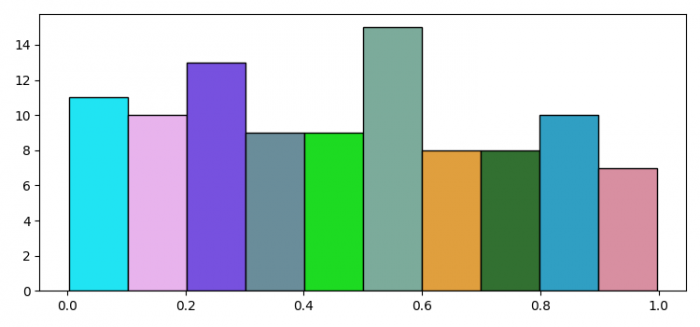# How to specify different colors for different bars in a Python matplotlib histogram?

To specify different colors for different bars in a matplotlib histogram, we can take the following steps −

## Steps

• Set the figure size and adjust the padding between and around the subplots.

• Create a figure and a set of subplots.

• Plot a histogram with random data with 100 sample data.

• Iterate in the range of number of bins and set random facecolor for each bar.

• To display the figure, use show() method.

## Example

import numpy as np
import matplotlib.pyplot as plt
import random
import string

# Set the figure size
plt.rcParams["figure.figsize"] = [7.50, 3.50]
plt.rcParams["figure.autolayout"] = True

# Figure and set of subplots
fig, ax = plt.subplots()

# Random data
data = np.random.rand(100)

# Plot a histogram with random data
N, bins, patches = ax.hist(data, edgecolor='black', linewidth=1)

# Random facecolor for each bar
for i in range(len(N)):
patches[i].set_facecolor("#" + ''.join(random.choices("ABCDEF" + string.digits, k=6)))

# Display the plot
plt.show()

## Output

It will produce the following output −